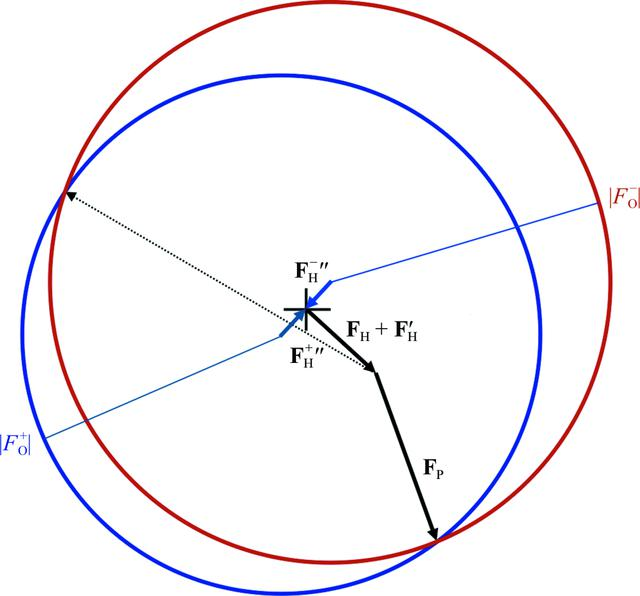disable zoom     view article Figure 2 The conventional Harker construction for SAD phasing. The total structure factors for F+ and F−* (the complex conjugate of F−) are sums of complex numbers (which can be represented as vectors) with common components. In the Harker construction we represent F+ as the vector sum of the imaginary contribution from the anomalous scatterers (FH+′′), the real contribution from the anomalous scatterers (FH + FH′) and the unknown contribution from the rest of the protein (FP, represented with two possibilities in solid and dashed arrows). Since the amplitude of the total structure factor, |Fo+|, is known, the FP vector must end up on the blue circle, which is centred on the tail of the FH+′′ vector and has a radius of |Fo+|. Similarly, F−* is represented as a vector sum, starting with its imaginary contribution from the anomalous scatterers (FH−′′) and then sharing the remaining real scattering components. The red circle, which is centred on the tail of the FH−′′ vector and with a radius of |Fo+|, crosses the blue circle at the two possible values for FP; the shorter of the two possible vectors is more probable. If the structure factor will be used for a map containing the anomalous scatterers, the origin of the Harker construction is taken at the base of the vector for the real contribution from the anomalous scatterers, indicated by a cross.BIOLOGICALCRYSTALLOGRAPHY
ISSN: 1399-0047
Volume 67| Part 4| March 2011| Pages 338-344